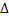Want to share your content on R-bloggers? click here if you have a blog, or here if you don't.

Have you ever wondered where do all those formulas to calculate the volume of solids like a cone, a cylinder, a sphere ecc… come from? In fact they come from a simple formula and from a clever basic idea. Imagine you have a function f

Intuitively you could approximate the volume by dividing your interval into a number n of small intervals of the same size and sum up the volume of the cylinders of radius f(x) and height, then

if we use an infinite number of steps n then our sum becomes an integral:

Now that we have the intuition, we  can go on to the code. I coded an example in R and one in Matlab. The one in Matlab is shorter since it deals with the integration on its own using symbolic computation while in R I decided to code a simple approximation algorithm based on the first formula I posted above, although you could easily calculate the indefinite integral of the function foo and then use it to calculate the exact volume.

Here is the Matlab example, quick, fast and neat:

Note that f(10)=5, thus the radius of our cone is 5. Furthermore, for the sake of simplicity I’ve assumed that the tip is in 0,0. This code outputs:

Here is the R code. Of course you could have made it shorter and more neat but I like coding in R so I made something more out of it. The R program below approximates the volume using the first approach described above.

Finally, you can get a 3D cone as the one at the top of this page just by using the RGL package in R and the demo scripts.